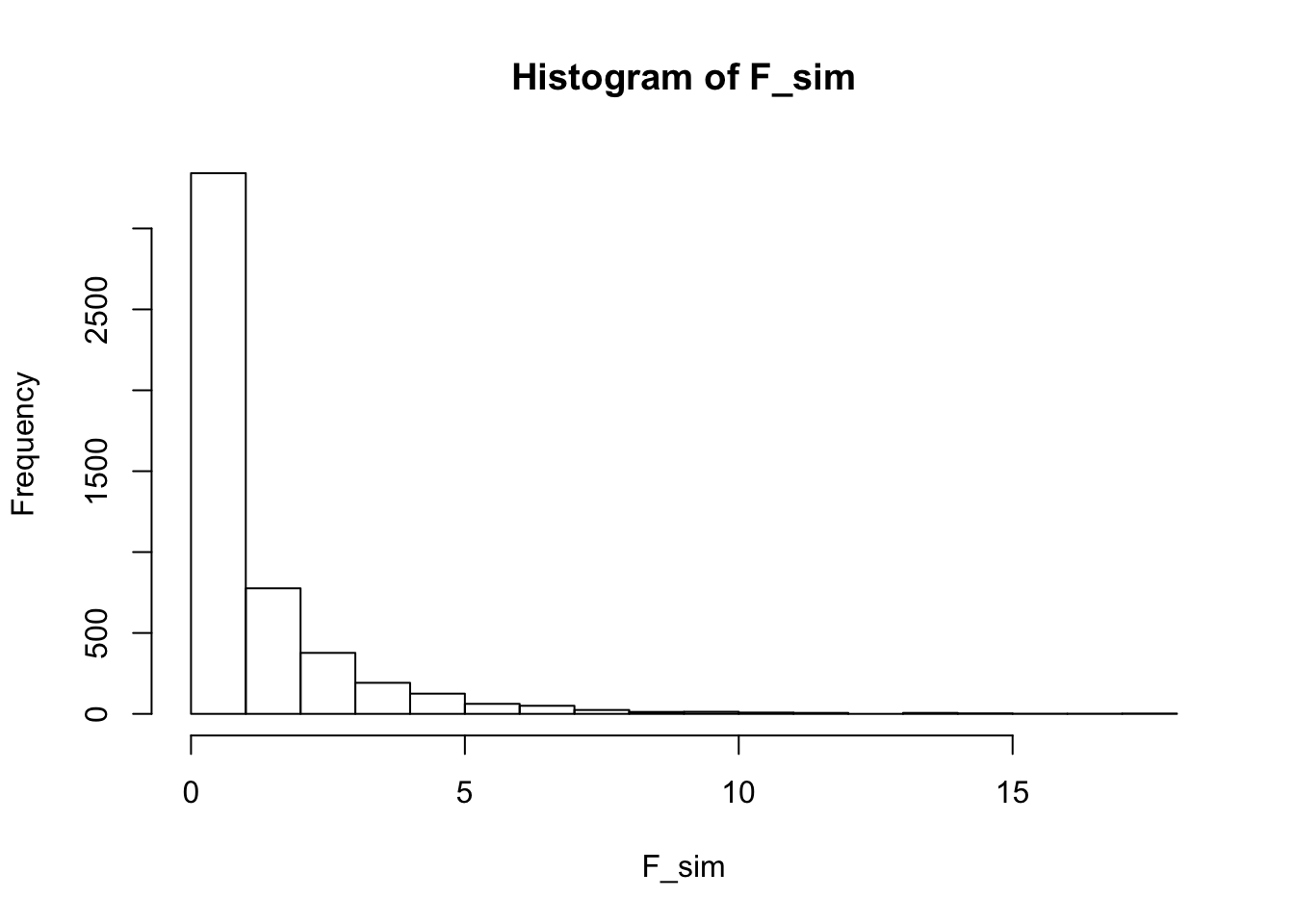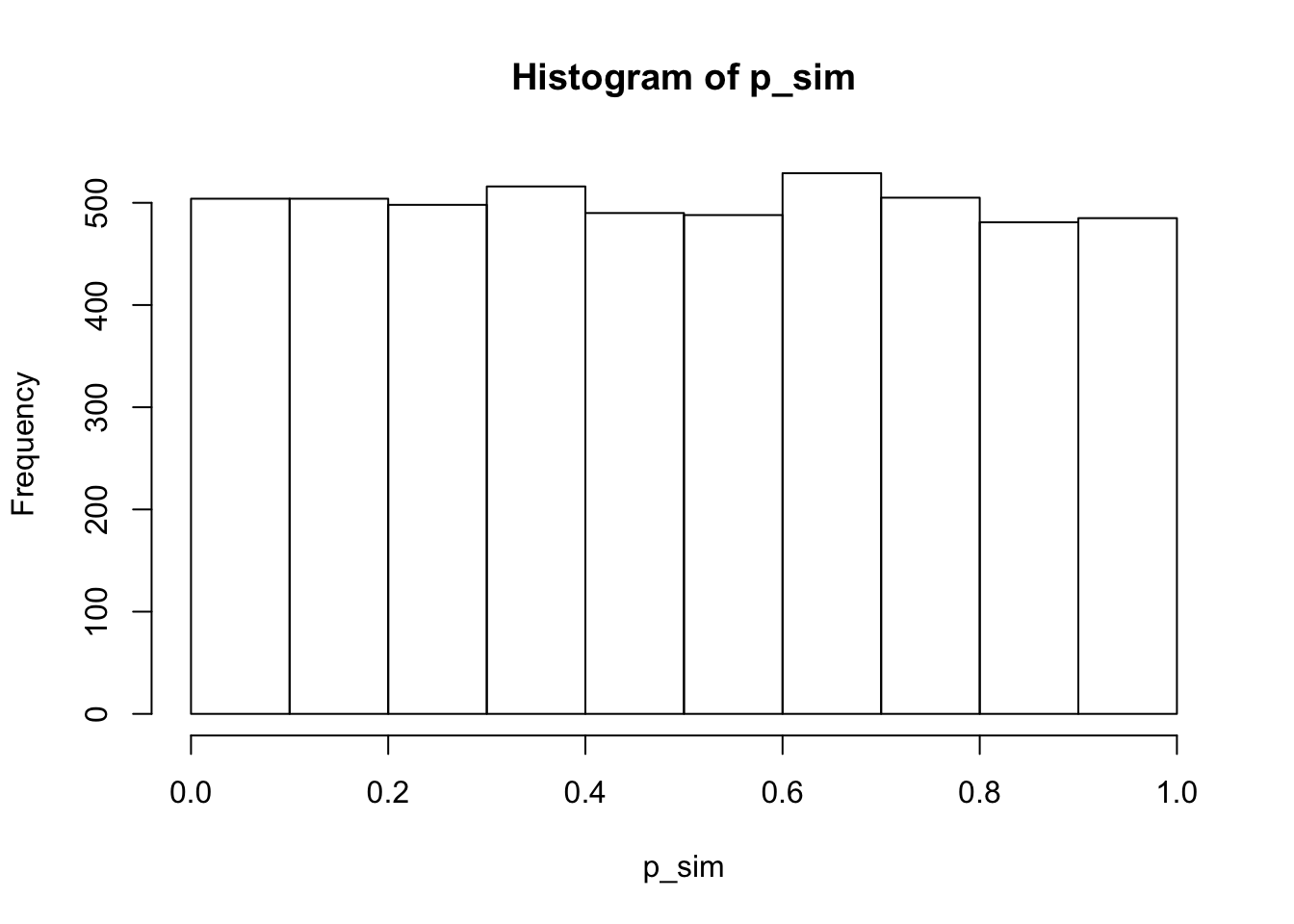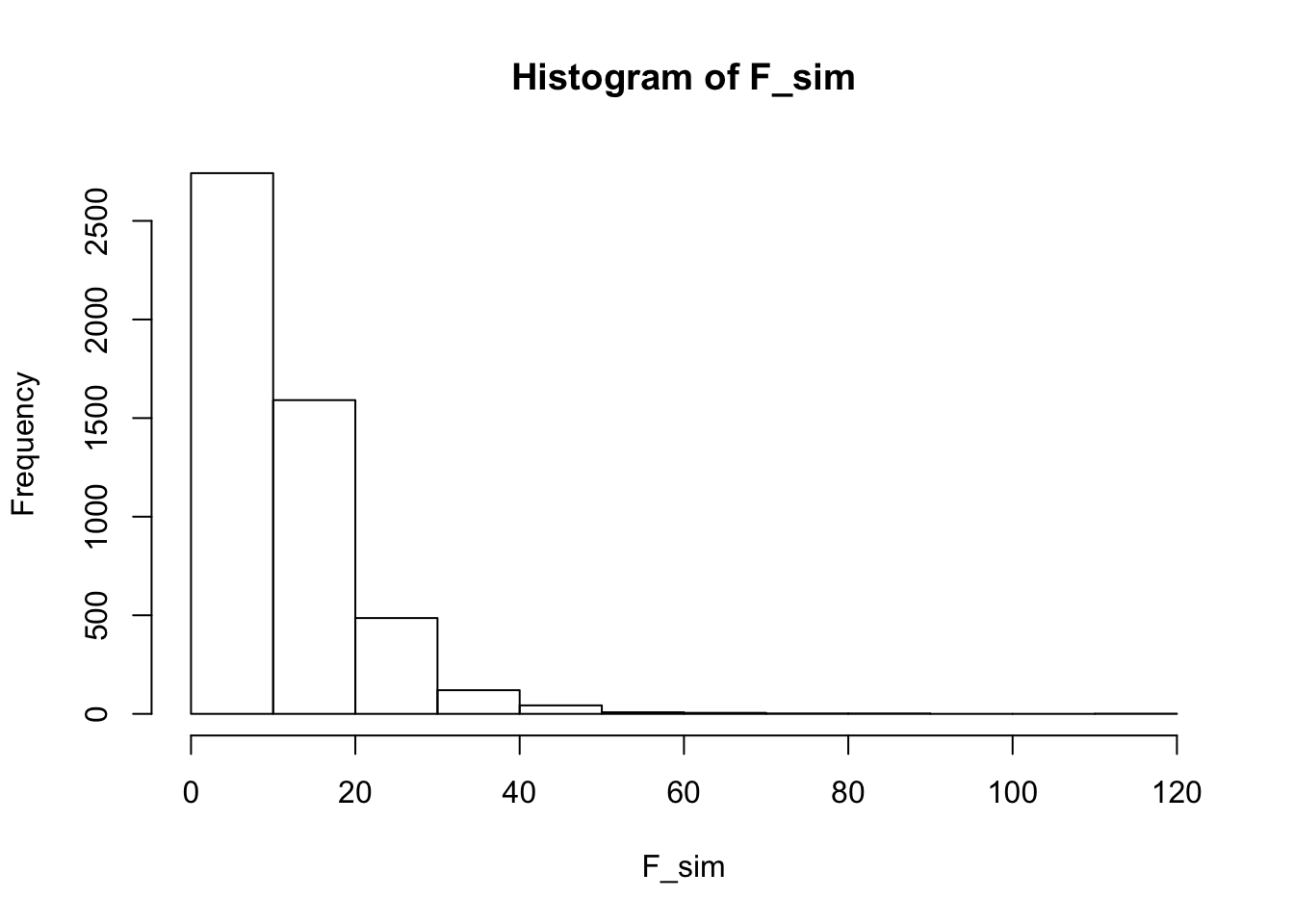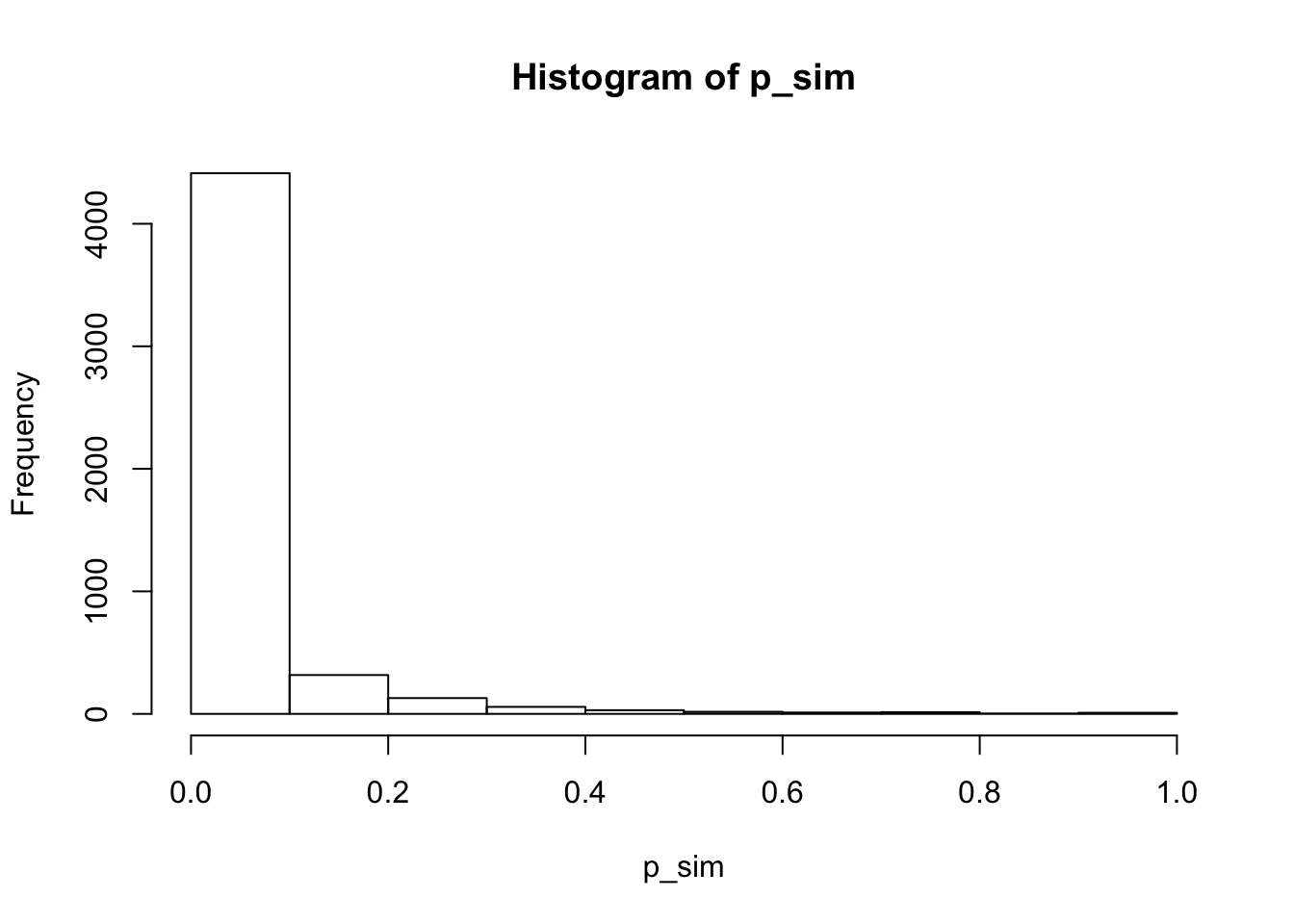Restricted and Full Models

x1 <- rnorm(n=20, mean=110, sd=15)
x2 <- rnorm(n=20, mean=100, sd=15)

Let’s compute the error for the full model:

E1 <- sum((x1-mean(x1))**2)
E2 <- sum((x2-mean(x2))**2)
Ef <- E1 + E2
df_f <- length(x1)+length(x2) - 2

Ef is 6286.8, df_f is 38.

Now let’s compute the error for the restricted model:

x_all <- c(x1,x2)
Er <- sum((x_all-mean(x_all))**2)
df_r <- length(x_all) - 1

Er is 8541.5, df_r is 39.

Now let’s compute F and lookup p:

F_obs <- ((Er-Ef)/(df_r-df_f)) / (Ef/df_f)
df1 <- df_r - df_f
df2 <- df_f
p_obs <- pf(F_obs, df1, df2, lower.tail=FALSE)

F_obs is 13.63 and p_obs is 0.000697.

Simulations

Let’s write a function that simulates an experiment.

gosim <- function(drugeffect, sampsize) {
x1 <- rnorm(n=sampsize, mean=100, sd=15)
x2 <- rnorm(n=sampsize, mean=100+drugeffect, sd=15)
E1 <- sum((x1-mean(x1))**2)
E2 <- sum((x2-mean(x2))**2)
Ef <- E1 + E2
df_f <- length(x1)+length(x2) - 2
x_all <- c(x1,x2)
Er <- sum((x_all-mean(x_all))**2)
df_r <- length(x_all) - 1
F_obs <- ((Er-Ef)/(df_r-df_f)) / (Ef/df_f)
df1 <- df_r - df_f
df2 <- df_f
p_obs <- pf(F_obs, df1, df2, lower.tail=FALSE)
return(list(F=F_obs, p=p_obs))
}

Simulate one experiment where the effect of the drug is zero, and we use a sample size of 20 subjects for each group (control and drug):

results <- gosim(0,20)
(results)
## $F ##  1.232694 ## ##$p
##  0.2738602

Simulating Type-I Error

Assuming the null hypothesis is true, and the drug has no effect, if we were to conduct our experiment 5000 times, how many times would we incorrectly reject the null hypothesis and conclude the drug had an effect?

n <- 5000
F_sim <- vector(mode="numeric", length=n)
p_sim <- vector(mode="numeric", length=n)
for (i in 1:n) {
results <- gosim(drugeffect=0, sampsize=10)
F_sim[i] <- results$F p_sim[i] <- results$p
}

Let’s draw a histogram of our F values and our p values:

hist(F_sim)hist(p_sim)You can see that most F values are small, and there is a uniform distribution of p values between 0 and 1.

How many p-values are below, say, 0.05?

typeIerrors <- length(which(p_sim<0.05))

There were 257 Type-I errors out of 5000, which corresponds to a probability of p=0.0514.

Simulating Type-II Error (and statistical Power)

Now let’s simulate experiments where the effect of the drug is non-zero (say +20) and count how many times out of 5000 simulated experiments we fail to (correctly) reject the null hypothesis. This will be our Type-II error. We can also compute statistical power as the number of times we correctly reject the null hypothesis. This is just 1 minus Type-II error.

n <- 5000
F_sim <- vector(mode="numeric", length=n)
p_sim <- vector(mode="numeric", length=n)
for (i in 1:n) {
results <- gosim(drugeffect=20, sampsize=10)
F_sim[i] <- results$F p_sim[i] <- results$p
}

Let’s draw a histogram of our F values and our p values:

hist(F_sim)hist(p_sim)You can see that while most F values are small the distribution is shifted to the right compare to our null hypothesis simulation above.

The distribution of p values is no longer uniformly distributed between 0 and 1, but is skewed to the lower values.

How many p-values are above 0.05? (These are Type-II Errors)

typeIIerrors <- length(which(p_sim>=0.05))

There were 1016 Type-II errors out of 5000, which corresponds to a probability of p=0.2032.

Thus our statistical power is (1-0.2032) which is 0.7968.